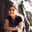RELATED TAGS

c++

# What is the copy-and-swap idiom in C++?Anjana Shankar

### The copy-and-swap idiom

In C++, a correct resource manager class needs to follow the rule of three. This rule states that if a class defines one of the three special functions (copy constructor, copy assignment, and destructor), it should explicitly define all these three functions.

Of these three functions, the copy assignment function is a bit nuanced. If that function fails, it may leave the original object in an inconsistent state, thus violating strong exception safety.

The copy-and-swap idiom lets us implement the copy assignment operator with a strong exception safety guarantee.

### Example

Let’s consider the implementation of a simple storage class. The class implements the constructor, copy constructor, copy assignment operator, and destructor.

#include <algorithm>
#include <memory>
#include <iostream>

class CharStore {
public:
CharStore(std::size_t size = 0):
sz(size),
store(std::make_unique<char[]>(sz)) {}
// Copy-constructor
CharStore(const CharStore& other):
sz(other.sz),
store(std::make_unique<char[]>(sz)){
std::copy(other.store.get(), other.store.get() + sz, store.get());
}
// Copy-assignment
CharStore& operator=(const CharStore& other) {
// 1. prevent self-assignment
if (this != &other) {
store.reset(); // 2. delete old data
// 3.0 prepare to assign new data
sz = other.sz;
// 3.1 create storage for assignment
store = std::make_unique<char[]>(sz);
// 3.2 assign new data
std::copy(other.store.get(), other.store.get() + sz, store.get());
}
return *this;
}

void copyStr(char const *p, size_t len) {
auto dst = store.get();
for (size_t i = 0; i < len; ++i) {
dst[i] = p[i];
}

}

void print() {
auto arr = store.get();
for (size_t i = 0; i < sz; ++i)
std::cout <<  arr[i];
std::cout << std::endl;
}

~CharStore() {}
private:
std::size_t sz;
std::unique_ptr<char[]> store;
};

int main()
{
CharStore src(14);
CharStore dst(14);
char const *a = "This is test";
char const *b = "That is test";
src.copyStr(a, 13);
dst.copyStr(b, 13);
src.print();
dst.print();

dst = src;
dst.print();

}
Simple storage class

### Explanation

• Line 5: We create a CharStore class. This toy class is responsible for storing some ASCII-text in its private variable store on line 50.
• Lines 7–9: We define a constructor.
• Lines 11-15: We define a copy constructor that constructs an object for the CharStore class from another existing object. Here, we copy the contents of the existing object’s store variable to the store of the new object.
• Lines 17–29: We define the copy-assignment operator. Here, we assign some object of the CharStore class to another object of the same class.
• Line 20: We delete the existing data of the store variable.
• Lines 22–24: We create sufficient storage to hold the new data.
• Line 26: We copy the contents from the source object’s store to the destination object’s store.

Note: This class does not provide strong exception safety. This is because if the statement at Line 24 fails, the existing object is modified. Another issue with this implementation is the repetition of the code between the copy constructor and the copy assignment operator.

The copy-and-swap idiom provides an elegant technique to avoid these problems. It utilizes the copy constructor to create a temporary object, and exchanges its contents with itself using a non-throwing swap. Therefore, it swaps the old data with new data. The temporary object is then destructed automatically (RAII).

### Correct solution

The following example illustrates the use of the copy-and-swap idiom to correctly implement the copy assignment operator to provide strong exception safety.

#include <algorithm>
#include <memory>
#include <iostream>

class CharStore {
public:
CharStore(std::size_t size = 0):
sz(size),
store(std::make_unique<char[]>(sz)) {}
// Copy-constructor
CharStore(const CharStore& other):
sz(other.sz),
store(std::make_unique<char[]>(sz)){
std::copy(other.store.get(), other.store.get() + sz, store.get());
}
// Copy-assignment
CharStore& operator=(const CharStore& other) {
CharStore temp(other);
temp.swap(*this);

return *this;
}
// Non-throwing swap function
void swap(CharStore& second) {
using std::swap;
swap(sz, second.sz);
swap(store, second.store);
}

void copyStr(char const *p, size_t len) {
auto dst = store.get();
for (size_t i = 0; i < len; ++i) {
dst[i] = p[i];
}

}

void print() {
auto arr = store.get();
for (size_t i = 0; i < sz; ++i)
std::cout <<  arr[i];
std::cout << std::endl;
}

~CharStore() {}
private:
std::size_t sz;
std::unique_ptr<char[]> store;
};

int main()
{
CharStore src(14);
CharStore dst(14);
char const *a = "This is test";
char const *b = "That is test";
src.copyStr(a, 13);
dst.copyStr(b, 13);
src.print();
dst.print();

dst = src;
dst.print();

}
Implementing the copy-and-swap idiom

### Explanation

Here, we modify the copy assignment operator as seen in lines 17–22.

• Line 18: We create a temp object from the assigned object using the copy constructor.
• Line 19: If the temp object is created successfully, we swap its content with the existing object using a non-throwing swap.
• Lines 24–28: We implement the swap function.

This example shows that the copy-and-swap idiom lets ys avoid code duplication in the copy-assignment operator by reusing the copy-constructor to create the temporary object.

Also, the state of the current object is not modified if the creation of the temporary object fails. The swap happens only after the temporary object is ready to use. This provides strong exception safety for the class above.

### Conclusion

The copy-and-swap idiom is an age-old technique used to implement the tricky copy-assignment operator. The benefits of strong exception safety are often useful, and the copy-and-swap idiom allows us to provide such a safety guarantee.

RELATED TAGS

c++

CONTRIBUTORAnjana Shankar
RELATED COURSES

View all Courses

Keep Exploring

Learn in-demand tech skills in half the time